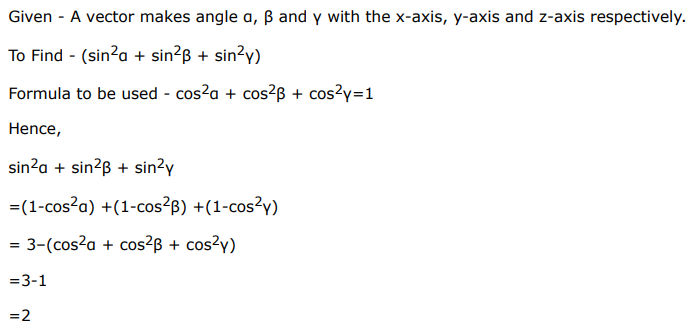# Mark against the correct answer in each of the following:

Question:

Mark $(\sqrt{)}$ against the correct answer in each of the following:

If a vector makes angle $a, \beta$ and $y$ with the $x$-axis, $y$-axis and $z$-axis respectively then the value of $\left(\sin ^{2} a+\sin ^{2} \beta+\sin ^{2} y\right)$ is

A. 1

B. 2

C. 0

D. 3

Solution: